# Examen détaillé des axicons

An axicon is a conical prism defined by its alpha (α) and apex angles. Unlike a converging lens (e.g. a plano-convex (PCX), double-convex (DCX), or aspheric lens), which is designed to focus a light source to a single point on the optical axis, an axicon uses interference to create a focal line along the optical axis (Figure 1). Within the beam overlap region (called the depth of focus, DOF), the axicon can replicate the properties of a Bessel beam, a beam comprised of rings equal in power to one another. The Bessel beam region may be thought of as the interference of conical waves formed by the axicon.1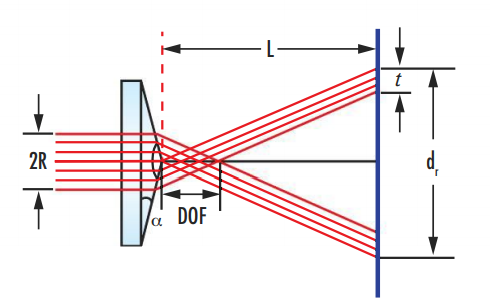### Bessel Beam Features of an Axicon

Unlike a Gaussian beam which deteriorates over distance, a Bessel beam is non-diffracting, maintaining an unchanged transversal distribution as it propagates. Although a true Bessel beam would require an infinite amount of energy to create, an axicon generates a close approximation with nearly non-diffracting properties within the Axicon's depth of focus (DOF). DOF is a function of the radius of the beam entering the axicon (R), the axicon's index of refraction (n), and the alpha angle (α):

(1)$$\text{DOF} = \frac{R \sqrt{1 - n^2 \sin^2 {\alpha} }}{\sin{\alpha} \cos{\alpha} \left( n \cos{\alpha} - \sqrt{1 - n^2 \sin^2 {\alpha}} \right)} \approx \frac{R}{\left( n - 1 \right) \alpha}$$

The simplified equation assumes that the angle of refraction is small and becomes less accurate as α decreases.

Beyond the axicon's depth of focus, a ring of light is formed. The thickness of the ring (t) remains constant and is equivalent to R:

(2)$$t = \frac{R \sqrt{1 - n^2 \sin^2 {\alpha}} }{\cos{\alpha} \left( n \sin^2 {\alpha} + \cos{\alpha} \sqrt{1-n^2 \sin^2 {\alpha}} \right) } \approx R$$

The simplified equation again assumes small angles of refraction. The diameter of the ring is proportional to distance; increasing length from lens output to image (L) will increase the diameter of the ring (dr), and decreasing distance will decrease it, as can be seen in Figure 1. As Equation 3 mathematically illustrates, the diameter of the ring is approximately related to twice the length, the tangent of the product of the refractive index (n), and the alpha angle (α).

(3)$$d_r = 2 L \left[ \frac{\sin{\alpha} \left( n \cos{\alpha} - \sqrt{1 - n^2 \sin^2 {\alpha}} \right) }{n \sin^2 {\alpha} + \cos{\alpha} \sqrt{1 - n^2 \sin^2 {\alpha}}} \right] \approx 2 L \tan{\left[ \left( n - 1 \right) \alpha \right]}$$

Figures 2 - 3 are real-world images of the focusing of green laser light by an axicon; the figures illustrate an axicon's ability to maintain constant ring thickness and proportional diameter with distance. In Figure 2, the axicon is positioned at L = 228.6mm and in Figure 3, at L = 355.6mm. The images were created using a laser with a beam diameter of 4mm, a 127mm x 127mm white balance target, and an axicon with a 20° alpha angle. For both Figure 2 and Figure 3, the thickness of the ring remained 2mm, while the diameter increased from approximately 73.66mm at L = 228.6mm between axicon output and image to approximately 114.3mm at L = 355.6mm.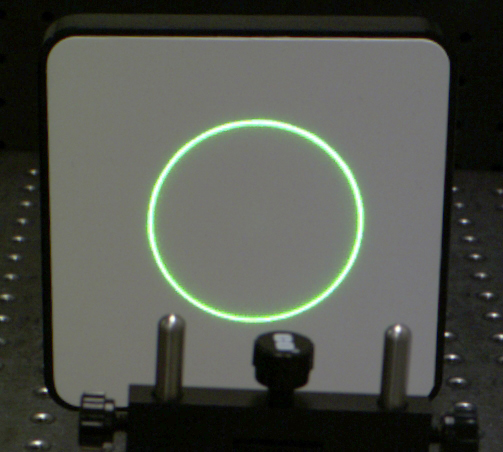##### Figure 2: Green laser light from an axicon at L = 228.6mm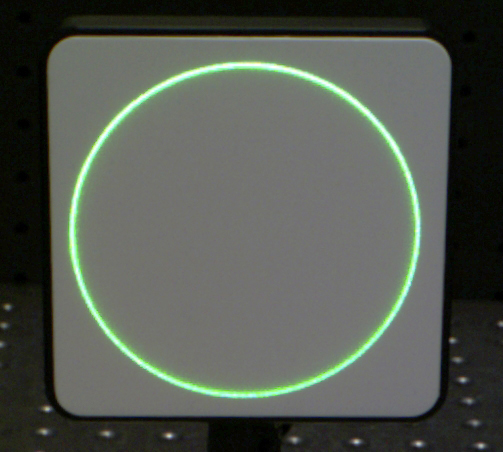### Applications of an Axicon

The unique properties of Bessel beams allow for axicon applications in a range of fields. Axicons can aid improvements in medical applications such as laser corneal surgery, in which the ring-shaped beam provides increased capability in smoothing and vaporizing the corneal tissue. With the use of a negative and positive axicon, ring diameter can be adjusted to fit the patient's and surgeon's needs by manipulating the distance between the two axicons.

Axicons are also beneficial in optical trapping, the use of a laser to create attractive and repulsive forces to manipulate microparticles and cells.2,3 The Bessel beam region within the DOF can trap particles on planar surfaces like a microscope slide without focal drift.2 The ring generated just beyond the DOF can also be used to isolate trapped objects.2

High-power Bessel beams, such as those formed by reflective axicons, are ideal for laser materials processing applications such as nano-channel drilling in glass (Figure 4).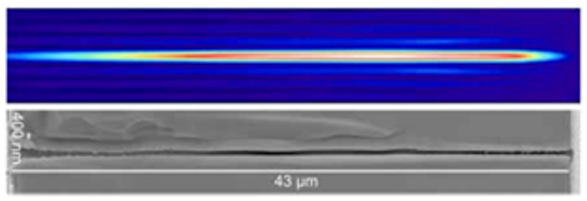### Using Optical Components with an Axicon

In order to replicate a Bessel beam, an axicon and laser must be aligned so that the laser beam travels along the axicon's optical axis. A variety of optical components can be used to achieve the alignment and precision needed to do this including lasers, beam expanders, optical lens mounts, and posts and post holders. For example, laser beam expanders collimate incident laser light and reduce its divergence so that an axicon can accurately create a ring-shaped beam, and optical lens mounts securely hold the axicon in place and can offer additional degrees of freedom for micron, or sub-micron, alignment.

By focusing a light source in a line along its optical axis, an axicon creates an approximation of a Bessel beam. Although the ring diameter increases and decreases proportionally to the distance between the axicon and the image, the ring thickness remains the same. Axicons are ideal for measurement and alignment, research, and medical applications requiring a ring-shaped laser output.

### Plano-Convex (PCX) Bessel Grade Axicons

• High-Precision Axicons
• 0.05° Apex Angle Tolerance
• Transform Gaussian Beams into Bessel Beam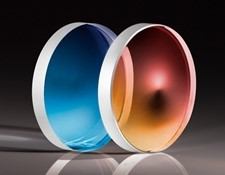VIEW NOW

### Plano-Convex (PCX) Ring Grade Axicons

• Lower Cost Than Bessel Grade Axicons
• Creates a Ring-Shaped Beam
• Multiple Apex Angles Available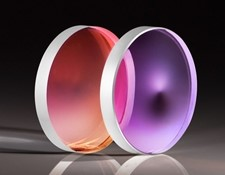VIEW NOW

References

More Resources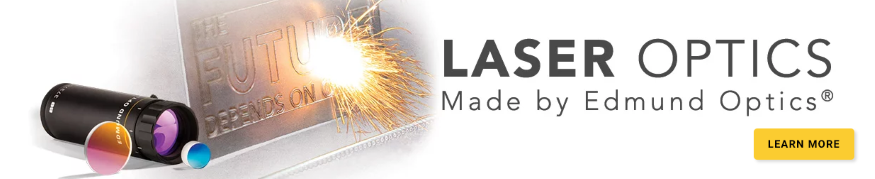Ce contenu vous a-t-il été utile ?